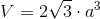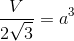## Example Questions

### Example Question #6 : Tetrahedrons

A regular tetrahedron has a surface area of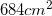. Each face of the tetrahedron has a height of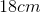. What is the length of the base of one of the faces?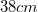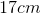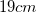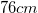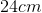Explanation:

A regular tetrahedron has 4 triangular faces. The area of one of these faces is given by: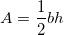Because the surface area is the area of all 4 faces combined, in order to find the area for one of the faces only, we must divide the surface area by 4. We know that the surface area is, therefore: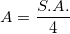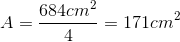Since we now have the area of one face, and we know the height of one face is, we can now plug these values into the original formula: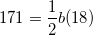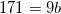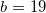Therefore, the length of the base of one face is.

### Example Question #7 : Tetrahedrons

What is the length of an edge of a regular tetrahedron if its surface area is 156?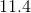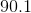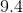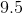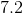Explanation:

The only given information is the surface area of the regular tetrahedron.

This is a quick problem that can be easily solved for by using the formula for the surface area of a tetrahedron: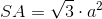If we substitute in the given infomation, we are left with the edge being the only unknown.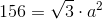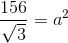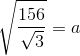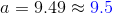### Example Question #8 : Tetrahedrons

What is the length of a regular tetrahedron if one face has an area of 43.3 squared units and a slant height of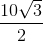?

Cannot be determined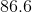Explanation:

The problem provides the information for the slant height and the area of one of the equilateral triangle faces.

The slant height merely refers to the height of this equilateral triangle.

Therefore, if we're given the area of a triangle and it's height, we should be able to solve for it's base. The base in this case will equate to the measurement of the edge. It's helpful to remember that in this case, because all faces are equilateral triangles, the measure of one length will equate to the length of all other edges.

We can use the equation that will allow us to solve for the area of a triangle: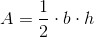whereis base length andis height.

Substituting in the information that's been provided, we get: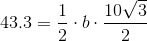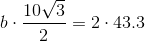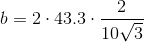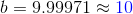### Example Question #9 : Tetrahedrons

The volume of a regular tetrahedron is 94.8. What is the measurement of one of its edges?

Cannot be determined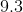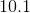Explanation:

This becomes a quick problem by just utilizing the formula for the volume of a tetrahedron.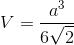Upon substituting the value for the volume into the formula, we are left with, which represents the edge length.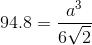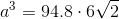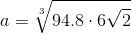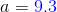### Example Question #10 : Tetrahedrons

A tetrahedron has a volume that is twice the surface area times the edge. What is the length of the edge? (In the answer choices,represents edge.)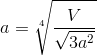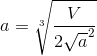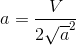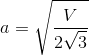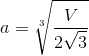Explanation:

The problem states that the volume is: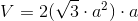The point of the problem is to solve for the length of the edge. Becasuse there are no numbers, the final answer will be an expression.

In order to solve for it, we will have to rearrange the formula for volume in terms of